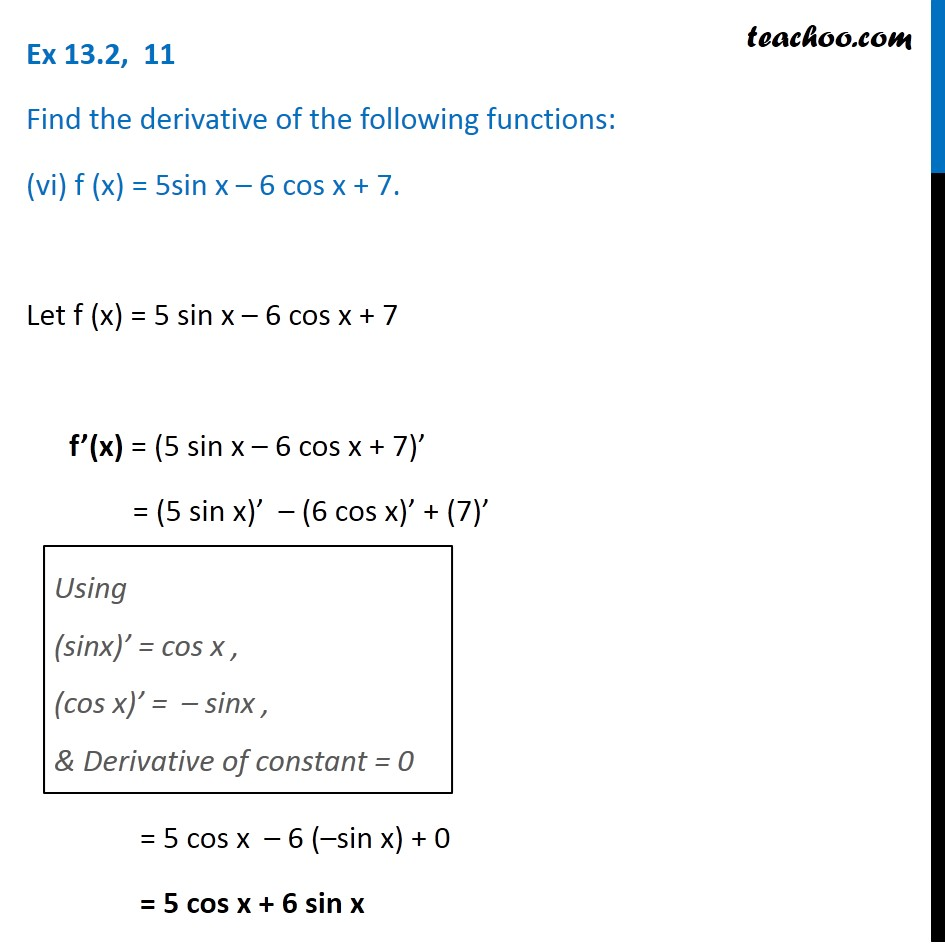Ex 12.2

Chapter 12 Class 11 Limits and Derivatives
Serial order wiseLearn in your speed, with individual attention - Teachoo Maths 1-on-1 Class

### Transcript

Ex 12.2, 11 Find the derivative of the following functions: (vi) f (x) = 5sin x – 6 cos x + 7. Let f (x) = 5 sin x – 6 cos x + 7 f’(x) = (5 sin x – 6 cos x + 7)’ = (5 sin x)’ – (6 cos x)’ + (7)’ Using (sinx)’ = cos x , (cos x)’ = – sinx , & Derivative of constant = 0 = 5 cos x – 6 (–sin x) + 0 = 5 cos x + 6 sin x